Design Three Phase Inverter using Simulink MATLAB

In this tutorial, you will learn how to design and simulate three phase voltage source inverter using Simulink MATLAB. I will explain you the working of inverters, more specifically 3 phase inverters. Inverters are one of the very basic circuits of electronics, also known as the opposite of rectifiers. At the start a comprehensive introduction of inverters as well as 3-phase inverters is provided along with the types of inverter and the effect of its types on the output. After that the circuit of a 3 phase inverter is implemented on MATLAB’s Simulink. We will also discuss the difference between both the types of inverters i.e. single phase and 3 phase  and compare the output of the block diagram constructed on Simulink. At the end a basic exercise is provided so that you can solve it on your own and it will strengthen your knowledge about inverters.

Introduction to Inverters

In electrical engineering inverters are one of the basic circuits used and are mostly used in UPS(Uninterrupted Power Supply) which is present in almost every house now a day. The basic purpose of an inverter is to convert the Direct Current (DC) to alternating current (AC), which is no doubt the opposite of rectifiers. For the beginners, the definition of AC voltage can be taken as the voltage which changes its direction from positive voltage to negative across same terminal in a specified period of time over and over again. A simple sinusoidal wave is an AC wave. However, DC can be defined as a constant source of voltage which remains either positive or negative through the course of time.

Types of Inverters

Inverters can be classified into two major types

• Single phase inverter.
• Three phase inverter.

Single phase inverter

Single phase inverter is the type of inverter in which only one DC source is used and the output thus formed is a single phase AC waveform. In the circuit, a bridge like circuit comprised of IGBT transistor is used which converts DC to AC

3 phase inverter

Alternatively, a three phase inverter uses two input DC sources, using 6 IGBT transistors to convert DC voltage into AC voltage and the output of such a circuit will be a three phase AC waveform with a phase difference of 120 . In the explanation below, we will design a three phase inverter in Simulink.

Design Three Phase Inverter using Simulink MATLAB

Open MATLAB and then open Simulink using the simulink icon on MATLAB as we have been doing in previous tutorials. Create a new blank model and save it in the first hand so you can access it in future. Now, click on the library browser icon on Simulink’s recently created model. In the library browser select the section named simscape as shown in the figure below,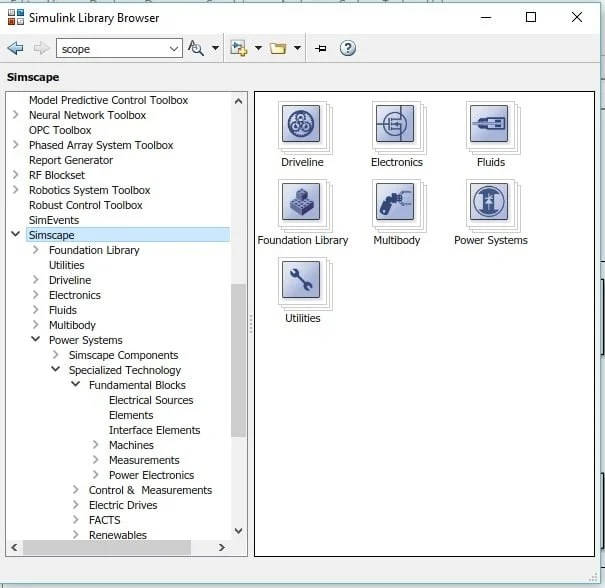Figure 1: Simscape

In this section select the block named power system as shown below,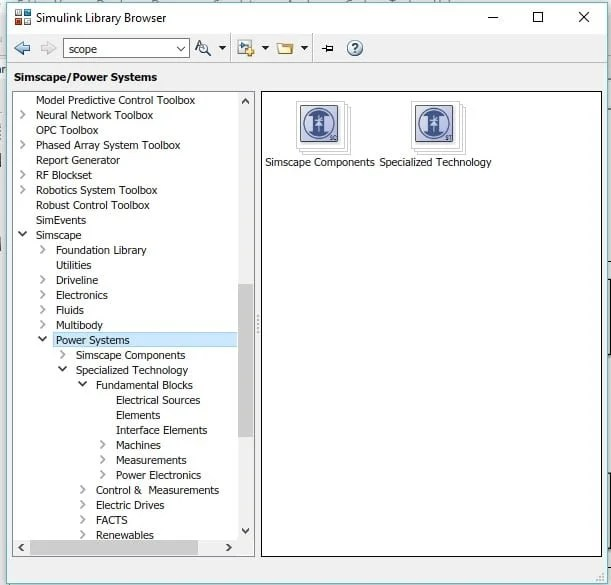Figure 2: Power system

From this section, now select Specialized Technology block as shown in the figure below,Figure 3: Specialized Technology block

Now go to the fundamental block present in this section as shown below,Figure 4: Fundamental blocks

In this section go to the power electronics block, in this block all the elements related power electronics are provided as shown in the figure below,Figure 5: Power electronics block

In an inverter as we know, thyristors are used in place of diodes (as in rectifiers). From here we will select the thyristor block named as IGBT / Diode as shown in the figure below,Figure 6: IGBT diodes

Place 6 such diodes on the model we created previously and arrange them in three rows having 2 thyristors each. Connect all of them to make a bridge as shown in the figure below,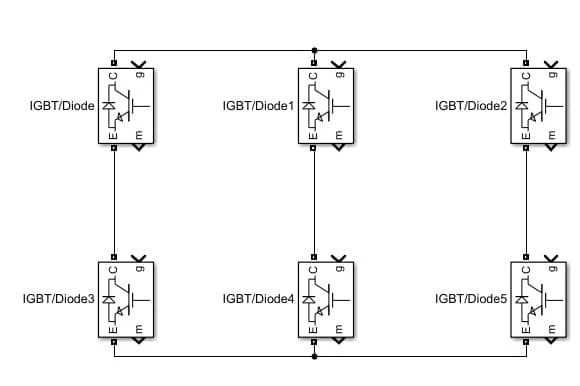Figure 7: Three phase bridge

Now double click on the thyristor block and in the block parameters dialog box, uncheck the point of showing measurable port as shown in the figure below,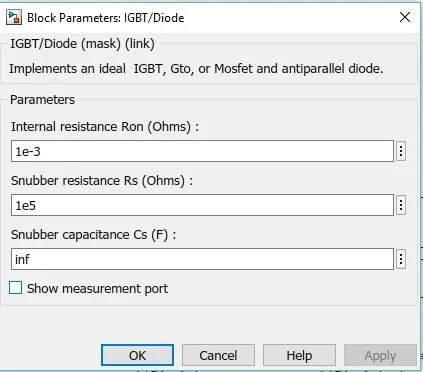Figure 8: Measurable port

In the library browser, go back to the fundamental block section as we have used before, and select the block named power gui and add it to the model as shown in the figure below,Figure 9: Power gui

This block contains the definition of all the power blocks used in simulink and acts like a startup file for the model. Now in the fundamental blocks section go to the sources section as shown in the figure below,Figure 10: Electrical sources

In this block select the DC source ( input of an inverter ) and add it to the model as shown in the figure below,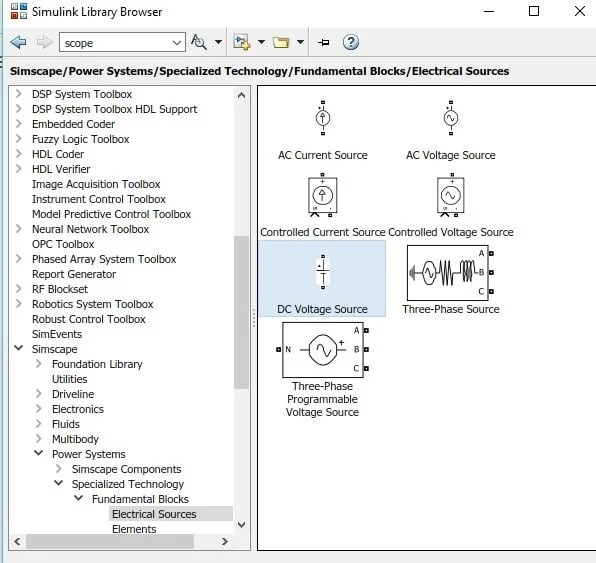Figure 11: DC source

To provide pulses to the thyristor we also need to place pulse generators to the model. In the library browser search for the pulse generator in the search bar and add the pulse generator to the model as shown in the figure below,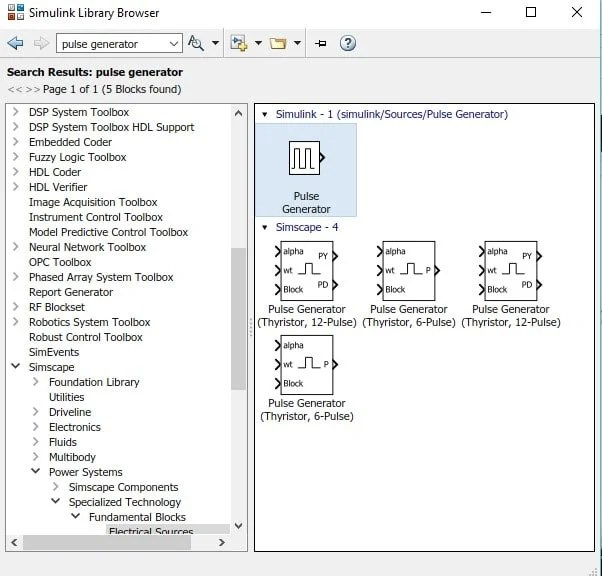Figure 12: Pulse generator

We need a different pulse generator for each of the thyristor hence place 6 different pulse generators for every thyristor. Now for the output side select the measurement block in the fundamental blocks section and in this section select the block named voltage measurement as shown in the figure below. As we are working with 3-phase inverter so we need to place three such measuring devices.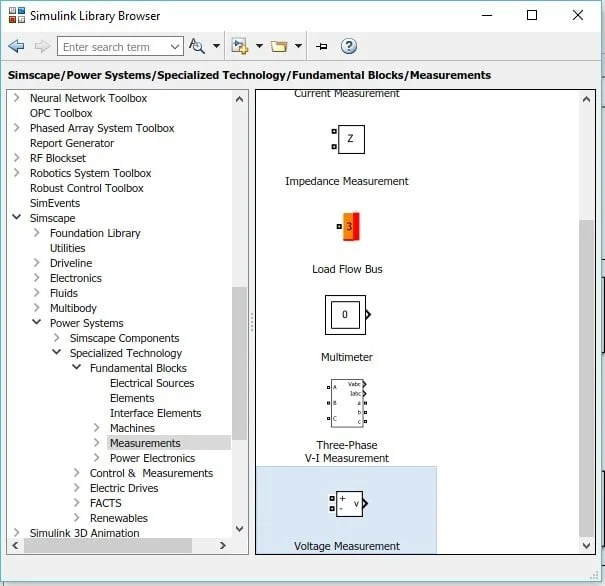Figure 13: Voltage measurement

Also we have to place the load to observe the output of he inverter. From the fundamental blocks section, select the block named elements and in this section select the series RLC branch and place it to the model as shown in the figure below,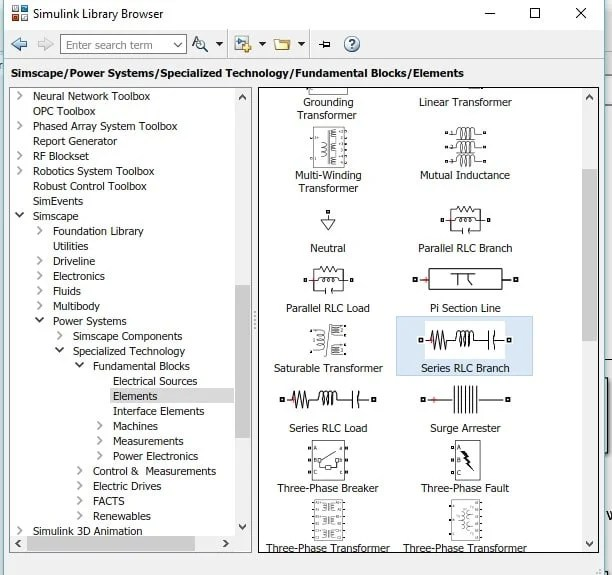Also in order to observe the output we need to place oscilloscope, place 3 scope blocks from the library browser on the model as shown in the figure below,Figure 15: Scope

The last thing to place is the ground, from the same section as of load, select the block named ground and place it in the model as shown in the figure below,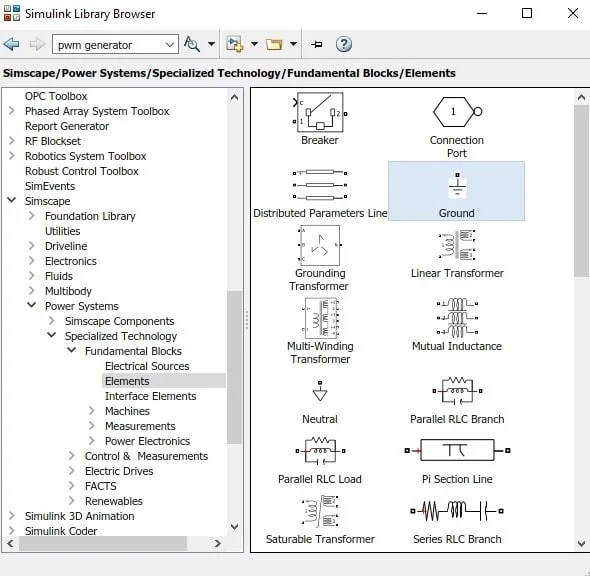Figure 16: Ground

At the input side of the bridge we created previously, connect the DC voltage source and at the output connect the load (change the RLC load to only resistive load) with each branch to each row as shown in the figure below,Figure 17: Load and source

Arrange all the 6 pulse generator with each of the thyristor and connect them. The phase shift of the first pulse generator will be 0 degrees and 60 degrees of the second and so on. The parameter settings of first block is shown in the figure below,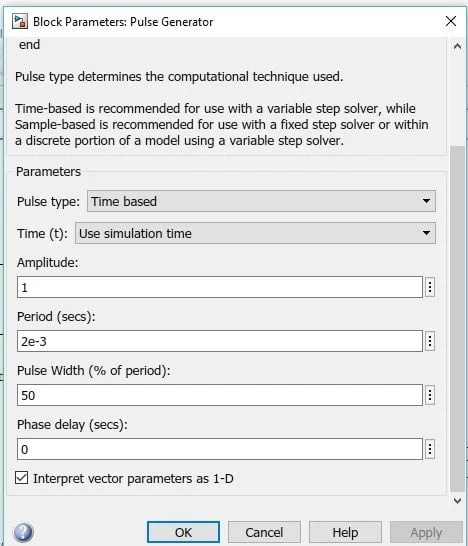Figure 18: Pulse generator settings of 1st pulse generator

As the phase delay is in seconds, so the phase delay of 2nd pulse generator will be (2e-3)*(1/6) and so on. All the six pulse generators connected are shown in the figure below,Figure 19: Pulse generator connected

At the output connect the voltage measurement across each resistor and connect the scope at the output of each voltmeter as shown in the figure below,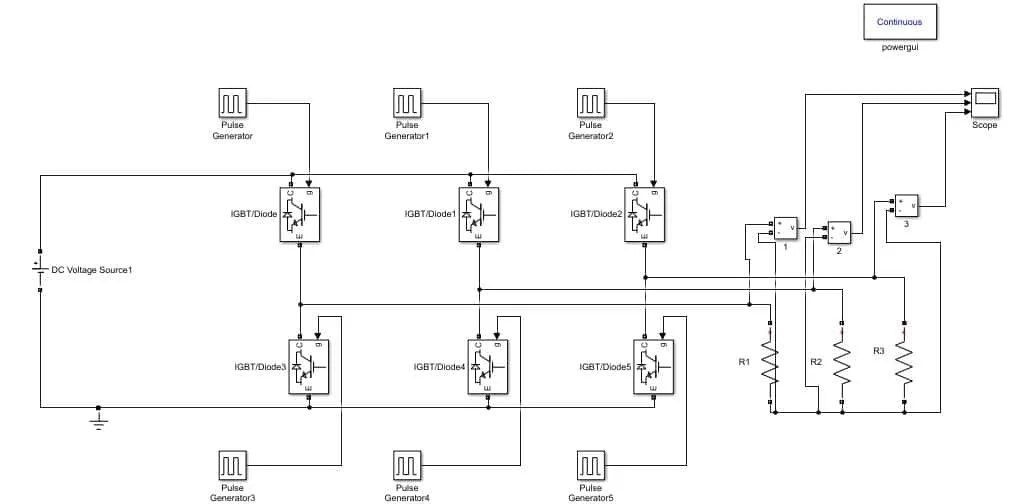Figure 20: Block diagram

Run the simulink model as we have been doing in previous tutorials and double click on the scope to see the output. The output will be a three phase AC as shown in the figure below,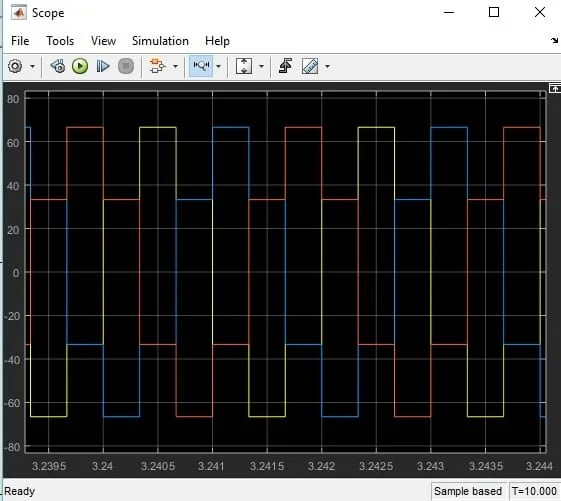Figure 21: Output of inverter

Change the layout of the scope to three frames and the output will look like as shown in the figure below,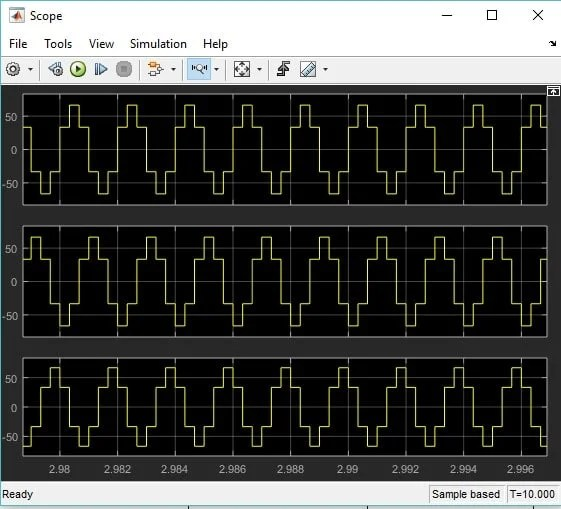Figure 22: Output of inverter

Exercise:

• Perform the analysis on single phase inverter using MATLAB’s Simulink.

(Hint: Number of transistor to be used will be 4)

1 thought on “Design Three Phase Inverter using Simulink MATLAB”

1. Tried this exact circuit, do not know what I am doing wrong but I do not get the same three phase waveforms at all. I have used the correct switching pulses and checked the waveforms but the output voltages are not right at all.